Скачать презентацию Summary Slide z Room Rate Structure z Hubbart

fb017b00deb2544b2bfccd053bcc34ea.ppt

• Количество слайдов: 15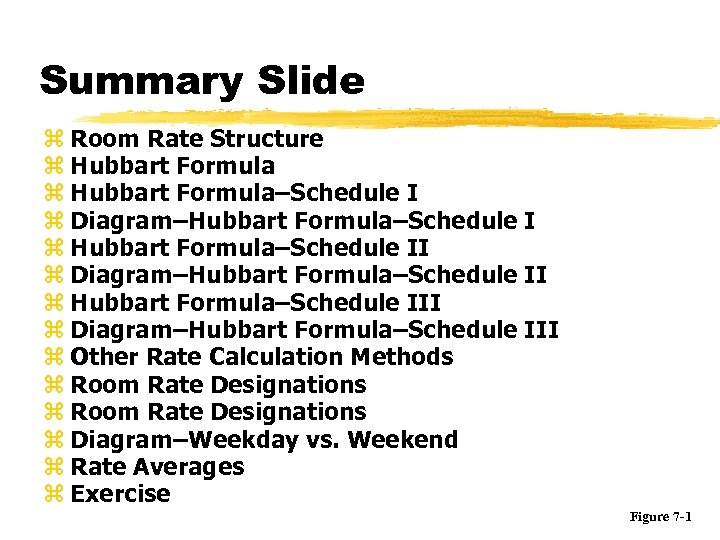Summary Slide z Room Rate Structure z Hubbart Formula–Schedule I z Diagram–Hubbart Formula–Schedule I z Hubbart Formula–Schedule II z Diagram–Hubbart Formula–Schedule II z Hubbart Formula–Schedule III z Diagram–Hubbart Formula–Schedule III z Other Rate Calculation Methods z Room Rate Designations z Diagram–Weekday vs. Weekend z Rate Averages z Exercise Figure 7 -1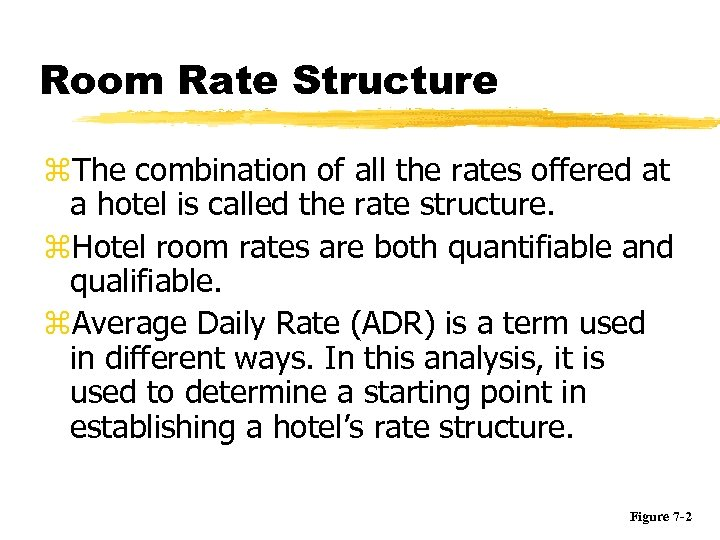Room Rate Structure z. The combination of all the rates offered at a hotel is called the rate structure. z. Hotel room rates are both quantifiable and qualifiable. z. Average Daily Rate (ADR) is a term used in different ways. In this analysis, it is used to determine a starting point in establishing a hotel’s rate structure. Figure 7 -2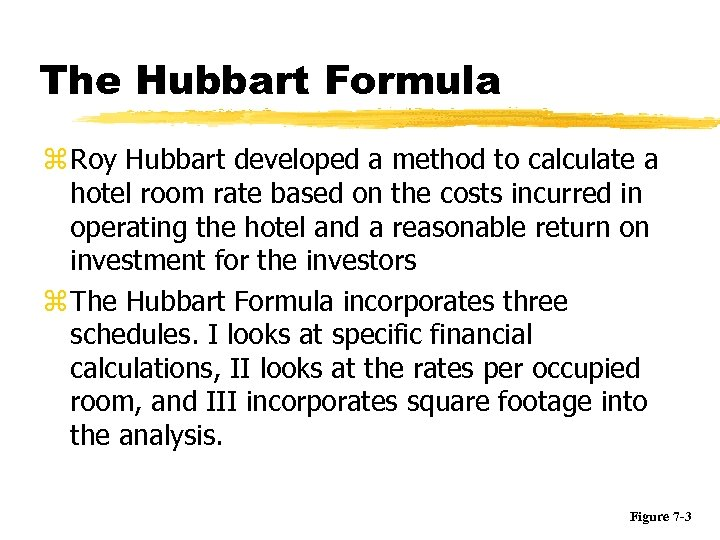The Hubbart Formula z Roy Hubbart developed a method to calculate a hotel room rate based on the costs incurred in operating the hotel and a reasonable return on investment for the investors z The Hubbart Formula incorporates three schedules. I looks at specific financial calculations, II looks at the rates per occupied room, and III incorporates square footage into the analysis. Figure 7 -3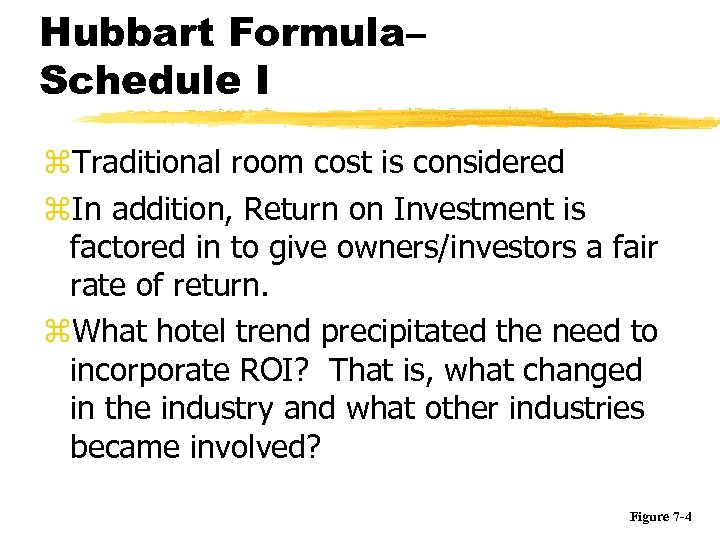Hubbart Formula– Schedule I z. Traditional room cost is considered z. In addition, Return on Investment is factored in to give owners/investors a fair rate of return. z. What hotel trend precipitated the need to incorporate ROI? That is, what changed in the industry and what other industries became involved? Figure 7 -4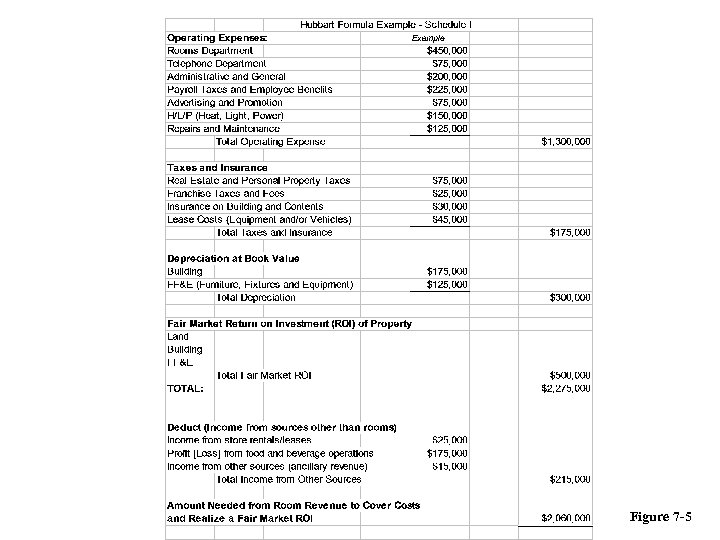Figure 7 -5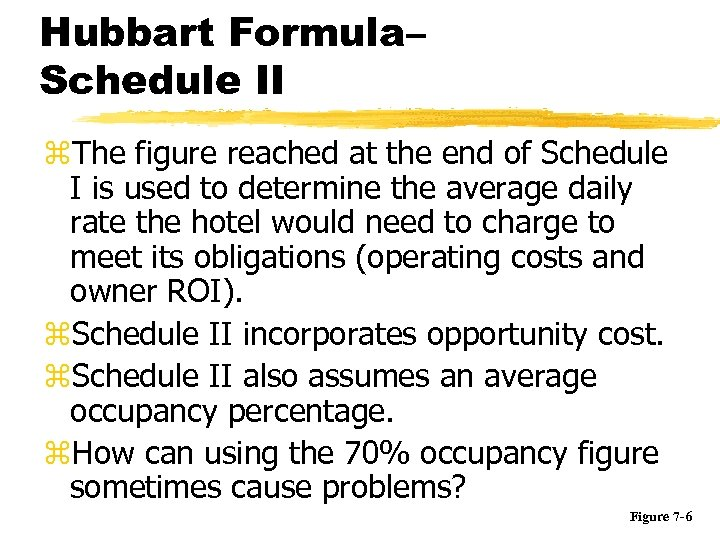Hubbart Formula– Schedule II z. The figure reached at the end of Schedule I is used to determine the average daily rate the hotel would need to charge to meet its obligations (operating costs and owner ROI). z. Schedule II incorporates opportunity cost. z. Schedule II also assumes an average occupancy percentage. z. How can using the 70% occupancy figure sometimes cause problems? Figure 7 -6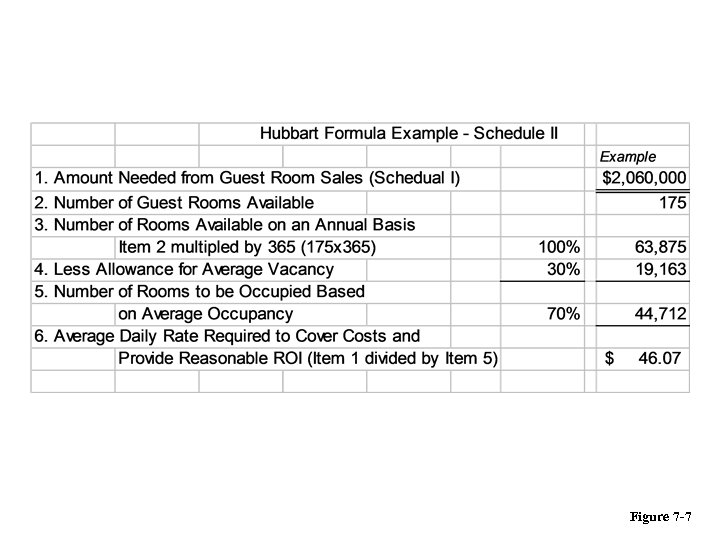Figure 7 -7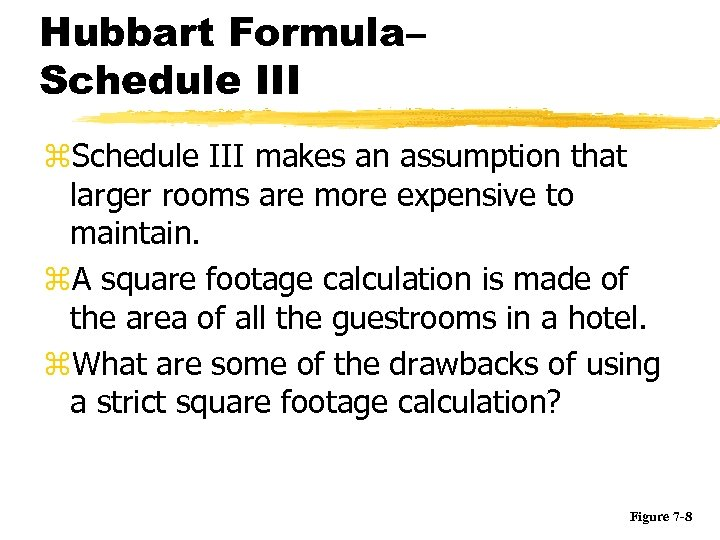Hubbart Formula– Schedule III z. Schedule III makes an assumption that larger rooms are more expensive to maintain. z. A square footage calculation is made of the area of all the guestrooms in a hotel. z. What are some of the drawbacks of using a strict square footage calculation? Figure 7 -8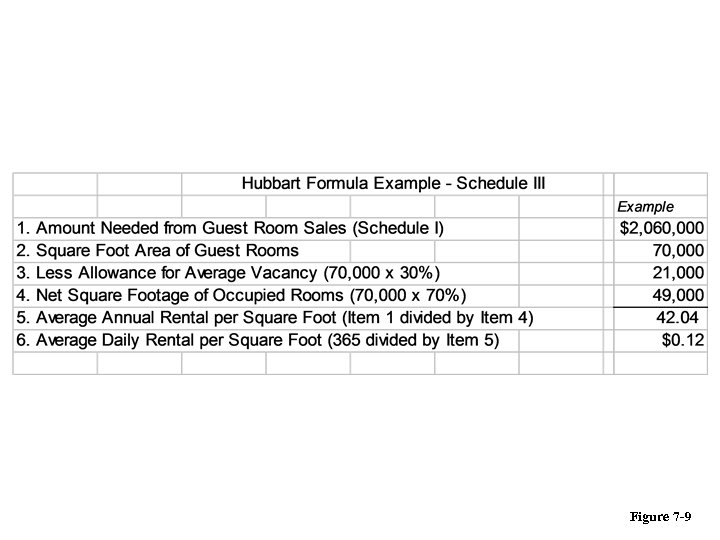Figure 7 -9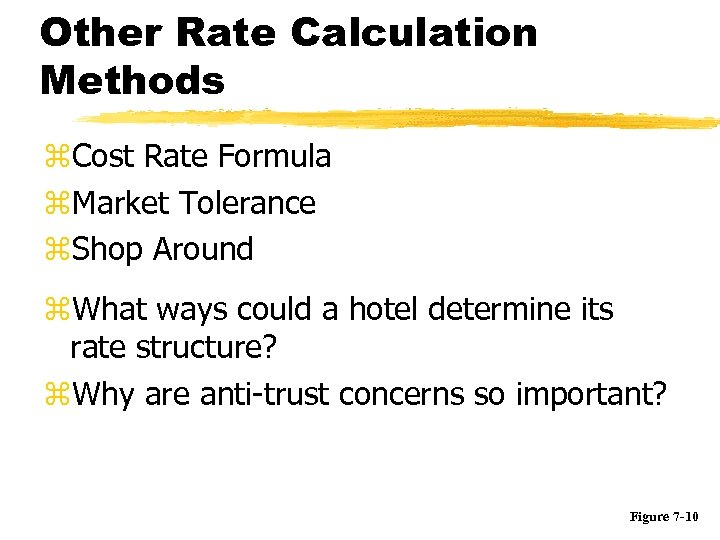Other Rate Calculation Methods z. Cost Rate Formula z. Market Tolerance z. Shop Around z. What ways could a hotel determine its rate structure? z. Why are anti-trust concerns so important? Figure 7 -10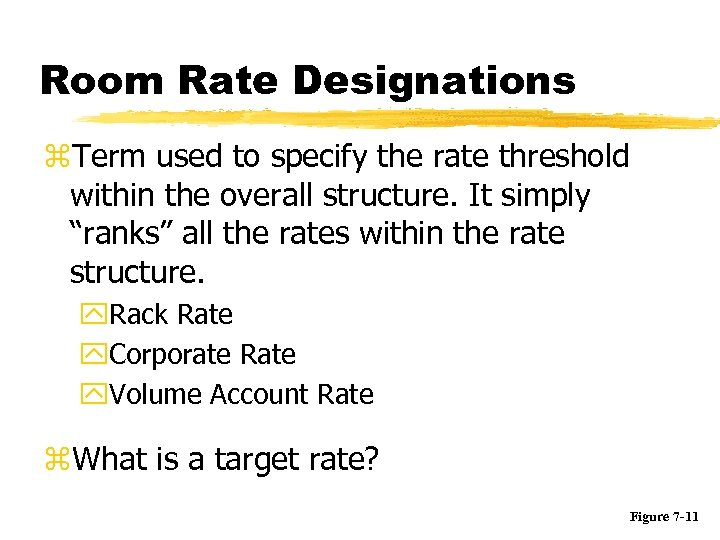Room Rate Designations z. Term used to specify the rate threshold within the overall structure. It simply “ranks” all the rates within the rate structure. y. Rack Rate y. Corporate Rate y. Volume Account Rate z. What is a target rate? Figure 7 -11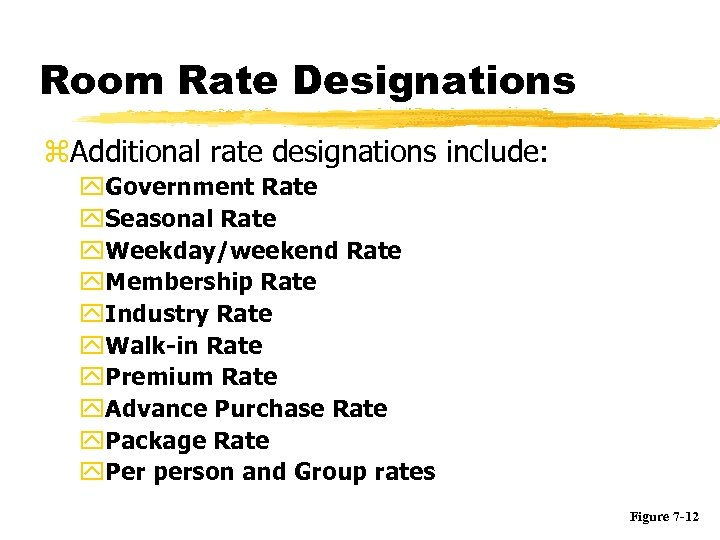Room Rate Designations z. Additional rate designations include: y. Government Rate y. Seasonal Rate y. Weekday/weekend Rate y. Membership Rate y. Industry Rate y. Walk-in Rate y. Premium Rate y. Advance Purchase Rate y. Package Rate y. Per person and Group rates Figure 7 -12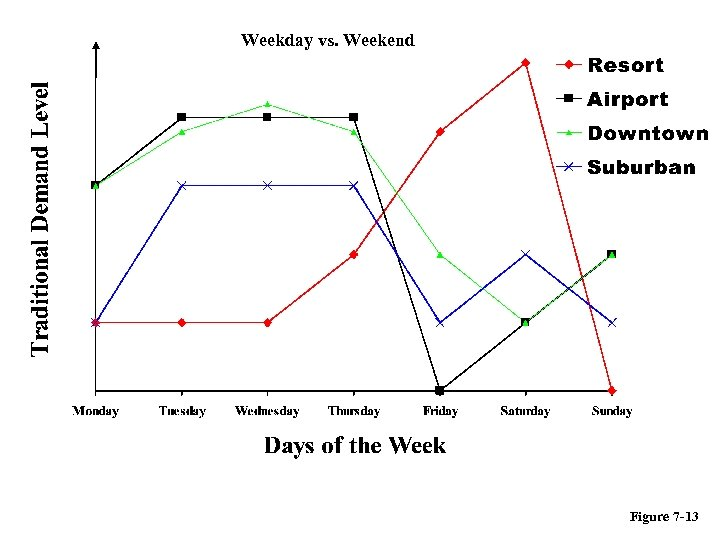Weekday vs. Weekend Figure 7 -13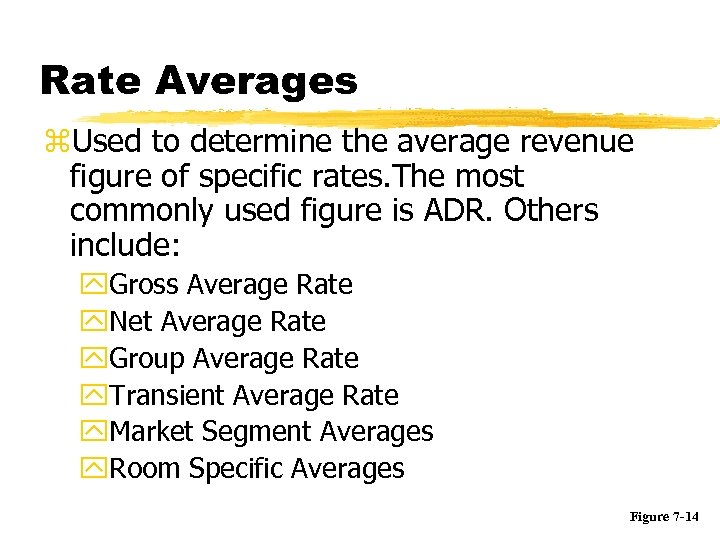Rate Averages z. Used to determine the average revenue figure of specific rates. The most commonly used figure is ADR. Others include: y. Gross Average Rate y. Net Average Rate y. Group Average Rate y. Transient Average Rate y. Market Segment Averages y. Room Specific Averages Figure 7 -14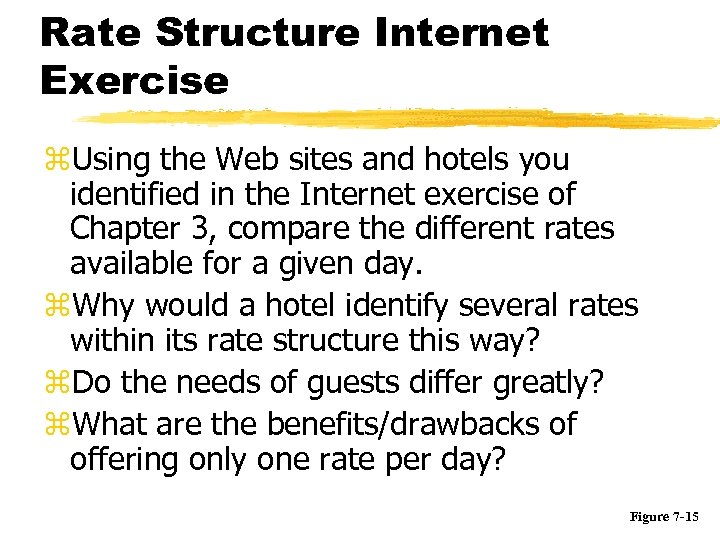Rate Structure Internet Exercise z. Using the Web sites and hotels you identified in the Internet exercise of Chapter 3, compare the different rates available for a given day. z. Why would a hotel identify several rates within its rate structure this way? z. Do the needs of guests differ greatly? z. What are the benefits/drawbacks of offering only one rate per day? Figure 7 -15